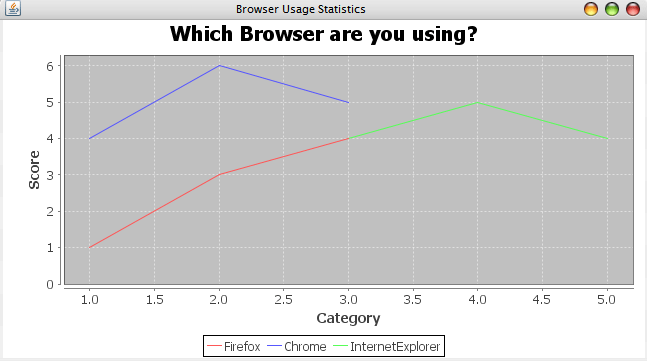# Create Line Chart Using JFreeChart

Create Line Chart Using JFreeChart explains about creating a simple line chart using JFreeChart API

A line chart or line graph is a type of chart which displays information as a series of data points connected by straight line segments. It is a basic type of chart common in many fields. It is similar to a scatter plot except that the measurement points are ordered (typically by their x-axis value) and joined with straight line segments

Reference -> http://en.wikipedia.org/wiki/Line_chart

How To Create Line Chart Using JFreeChart library?

JFreeChart is a free and open source java chart library used for creating professional quality charts. JFreeChart is purely written in java language, we can very easily incorporate JFreeChart in our java standalone and web applications.

Referenece - > http://www.jfree.org/jfreechart/

Here I am showing an example about How to create  a simple Line Chart using JFreeChart.

Note

1) If you need pie chart example, You can follow Create Pie Chart Using JFreeChart

2) If you need line chart example, You can follow Create Line Chart Using JFreeChart

3) If you need bar chart example, You can follow Create Bar Chart Using JFreeChart

4) If you need polar chart example, You can follow Create Polar Chart Using JFreeChart

5) If you need bubble chart example, You can follow Create Bubble Chart Using JFreeChart

6) If you need wind chart example, You can follow Create Wind Chart Using JFreeChart

###### Required Libraries

Following jar must be in classpath

1. jfreechart-1.5.0.jar
2. jcommon-1.0.24.jar

### pom.xml

```<dependency>
<groupId>org.jfree</groupId>
<artifactId>jfreechart</artifactId>
<version>1.5.0</version>
</dependency>
<dependency>
<groupId>org.jfree</groupId>
<artifactId>jcommon</artifactId>
<version>1.0.24</version>
</dependency>```

We can create following professional quality charts by using jfreechart.

• Single valued Charts such as compass, speedometer, thermometer
• Line Chart
• Pie Chart
• Bar Chart
• Bubble Chart
• Wind Chart
• Polar Chart

## JFreeChart Example(Line Chart)

import javax.swing.JFrame;

import org.jfree.chart.ChartFactory;
import org.jfree.chart.ChartPanel;
import org.jfree.chart.JFreeChart;
import org.jfree.chart.plot.PlotOrientation;
import org.jfree.data.xy.XYDataset;
import org.jfree.data.xy.XYSeries;
import org.jfree.data.xy.XYSeriesCollection;

public class JFreeChartLineChartExample extends JFrame {

private static final long serialVersionUID = 1L;

public JFreeChartLineChartExample(String applicationTitle, String chartTitle) {

super(applicationTitle);

// based on the dataset we create the chart

JFreeChart pieChart = ChartFactory.createXYLineChart(chartTitle, "Category", "Score", createDataset(),PlotOrientation.VERTICAL, true, true, false);

// Adding chart into a chart panel

ChartPanel chartPanel = new ChartPanel(pieChart);

// settind default size

chartPanel.setPreferredSize(new java.awt.Dimension(500, 270));

setContentPane(chartPanel);

}

private XYDataset createDataset() {

final XYSeries firefox = new XYSeries("Firefox");
(1.0, 1.0);
(2.0, 3.0);
(3.0, 4.0);

final XYSeries chrome = new XYSeries("Chrome");
(1.0, 4.0);
(2.0, 6.0);
(3.0, 5.0);

final XYSeries iexplorer = new XYSeries("InternetExplorer");
(3.0, 4.0);
(4.0, 5.0);
(5.0, 4.0);

final XYSeriesCollection dataset = new XYSeriesCollection();
(firefox);
(chrome);
(iexplorer);

return dataset;

}

public static void main(String[] args) {

JFreeChartLineChartExample chart = new JFreeChartLineChartExample("Browser Usage Statistics", "Which Browser are you using?");
chart.pack
();
chart.setVisible
(true);

}
}
###### Output1 Responses to "Create Line Chart Using JFreeChart"
1. Mustafa Turhan Coban 2018-02-19 06:11:50.0